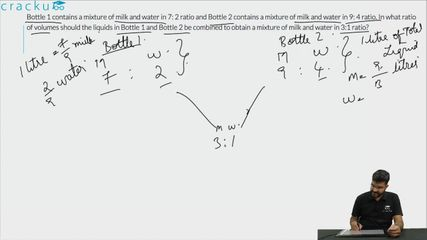Question 69

# Bottle 1 contains a mixture of milk and water in 7: 2 ratio and Bottle 2 contains a mixture of milk and water in 9: 4 ratio. In what ratio of volumes should the liquids in Bottle 1 and Bottle 2 be combined to obtain a mixture of milk and water in 3:1 ratio?

Solution

The ratio of milk and water in Bottle 1 is 7:2 and the ratio of milk and water in Bottle 2 is 9:4
Therefore, the proportion of milk in Bottle 1 is $$\frac{7}{9}$$ and the proportion of milk in Bottle 2 is $$\frac{9}{13}$$

Let the ratio in which they should be mixed be equal to X:1.

Hence, the total volume of milk is $$\frac{7X}{9}+\frac{9}{13}$$
The total volume of water is $$\frac{2X}{9}+\frac{4}{13}$$
They are in the ratio 3:1

Hence, $$\frac{7X}{9}+\frac{9}{13} = 3*(\frac{2X}{9}+\frac{4}{13})$$
Therefore, $$91X+81=78X+108$$

Therefore $$X = \frac{27}{13}$$

### View Video Solution• All Quant CAT Formulas and shortcuts PDF
• 30+ CAT previous papers with solutions PDF

##### Khyathi

7 months ago

Why u took x:1 as ratio

##### Mohit Goyal

1 week, 4 days ago

Same question